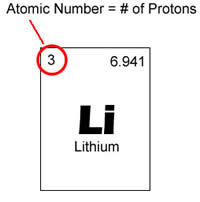Difference between Atomic mass and Atomic number

Key Difference: Atomic mass is simply the mass of a specific isotope, or the combined mass of the atom’s protons, neutrons and electrons. Atomic number is the number of protons that is found in a nucleus of an element.

Let’s face it chemistry is confusing, the various amount of elements, atomic numbers, bonds, mass, weight, atoms, etc. All these words start to blur after a certain amount of time that a person can no longer distinguish one from the other. Well atomic mass and atomic number are two of such words that are often confused and used interchangeably.Atomic mass is simply the mass of a specific isotope. An isotope is a variant of a particular chemical element. All the isotopes share the same number of protons, but the number of neutrons differs in each isotope. The mass of an atom includes the combined mass of the atom’s protons, neutrons and electrons. The atomic mass is sometimes incorrectly used to describe relative atomic mass, average atomic mass and atomic weight. However, they differ from atomic mass slightly.

The atomic mass is the mass of an atom when it is at rest. This is because when an atom is moving at a very high velocity, it tends to increase in mass. Though according to the definition, the atomic mass is the mass of all protons, neutrons and electrons, electrons are too small to make any significant difference in the atomic mass. Most of the elements on the periodic table have isotopes and due to the difference in the neutron count, the mass of each isotope also changes.

The atomic mass is expressed using atomic mass units or amu. One atomic mass unit is equal to 1/12th of the mass of Carbon-12. When the mass of an atom is divided by the 1/12th of the mass of Carbon-12, the relative mass of the element is obtained. However, relative mass of an object is actually the atomic weight of an element, as it is calculated by considered all of the isotopes. Though, this should not be assumed as atomic mass of an element.Atomic number is the number of protons that is found in a nucleus of an element. When an atom is neutral, it means that it has the same number of protons and electrons. Atomic number is denoted with a Z. Since each element has a different number of protons, this number is also used to represent an element. Isotopes usually share the same atomic number but are classified using their atomic mass, for example Carbon-12, Carbon-13.

Elements are placed in order of atomic number on a periodic table. The atomic number is listed in the left upper-corner of the element as a superscript. Atomic numbers can help divulge information about the element and many people can see the atomic number and state what element it is. It also tells where in the periodic table it can be placed and whether it is liquid, gas or solid. Atomic number also gives information regarding nucleus charge, oxidation states, bonding behavior, charge of the ion, etc.

Image Courtesy: happpyhalogens.blogspot.com, sawyerscience.com